6.6 Polygons (Rectilinear figures):

Let us look at the figures given below and observe their properties.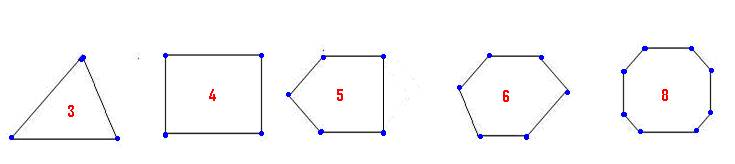They are on the same plane(surface)

They are closed figures

They are bounded by 3 or more lines.

They have 3 or more intersecting points.

Definition:

‘Polygons’ (figures with many sides) are figures with three or more line segments which are coplanar (lie on the same plane), non – collinear and

whose line segments intersect each other at their end points. The end points where line segments meet are called ‘vertices’ of the polygon.

The line segments which make the polygon are called ‘sides’ of the polygon.

The line segment joining any 2 non consecutive (not next to each other) vertices of a polygon

is called a ‘diagonal’. The adjacent figure has five sides and hence it is called pentagon.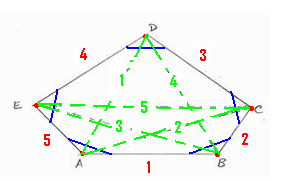Verticies(5) Sides (5) Angles (5) Diagonals(5) A, B,C,D,E AB, BC,CD,DE,EA ABC,BCD,CDE,DEA,EAB AD, AC, BE, BD, CE

Observation:

In a polygon, number of sides= number of angles = number of vertices.

Examples of polygons are Triangle (3 sides), Quadrilateral (4 sides), Pentagon (5 sides), Hexagon (6 sides), Heptagon (7 sides), Octagon (8 sides) and so on…

Definition:

1. A ‘regular polygon’ is a polygon which is both equilateral (all sides equal) and equiangular (all angles same). (Square is a best example of a

regular polygon as its sides are equal and angles are all equal to 900)

2. The ‘interior region’ of a polygon is a part of the plane enclosed by the polygon. (the space which is called area)

Exercise:

1. Draw a regular pentagon – do you notice that all interior angles are equal to 1080?

2. Draw a regular hexagon – do you notice that all interior angles are equal to 1200?

An ‘inscribed regular polygon’ is a regular polygon whose vertices are on a circle.

(Alternatively we can say that an ‘inscribed regular polygon’ is a regular polygon whose sides are chords (arcs) of a circle)

As an example let us look at a regular quadrilateral:

(From each of the vertices of the regular polygon draw lines to the center of the circle)

 1. Number of lines connecting to origin of the circle is equal to number of sides of polygon(In the adjacent figure 4 sides: AO, BO,CO,DO )   2. Number of angles formed at the origin of the circle is equal to number of sides of polygon( In the adjacent figure 4 angles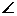AOB,BOC,COD,DOA) 3. Angles at the origin are equal to each other(=90o)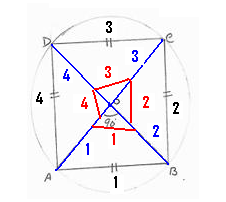6.6 Example 1: Construct an inscribed regular quadrilateral in a circle of radius 4cm:

 Step 1: Divide 3600 by the number of sides of the quadrilateral (=4) to get the angle at origin. Therefore angle at origin = 900 = 360/4.   Step 2: With O as center draw a circle of radius 4cm.   Step 3: Draw 2 lines (OA and OB) from O such thatAOB =90 0.(arrived in Step 1)   Step 4: With B as center cut an arc of radius =AB to cut the circle at C.   Step 5: With C as center cut an arc of radius = BC to cut the circle at D.   Step 6: Join the points A, B, C and D to get the quadrilateral ABCD.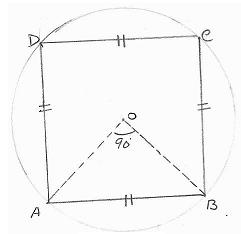General Method for constructing an inscribed regular polygon in a circle of a given radius:

 Step 1: Divide 3600 by the number of sides of the polygon to get the angle at origin. Angle at origin = 360/(No of sides of Polygon)   Step 2: With O as center draw a circle of given radius.   Step 3: Draw 2 lines (OA and OB) from O with the angle between them = Angle at origin (arrived in Step 1).   Step 4: With B as center cut an arc of radius=AB to cut the circle at C.   Step 5: With C as center cut an arc of radius =BC to cut the circle at D.   Step 6: Repeat step 5 till the last arc cuts the circle again at A.   Step 7: Join the points to get the required polygon.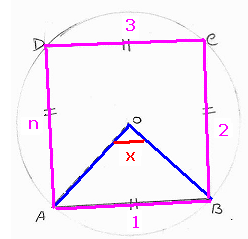Observations:

Angle at origin for different inscribed regular polygons:

 Polygon type No of sides Angle at center Triangle 3 1200(360÷3) Quadrilateral 4 900(360÷4) Pentagon 5 720(360÷5) Hexagon 6 600(360÷6) Octagon 8 450(360÷8)

Think why inscribed polygon of 7 sides is not included in the above table!

6.6.1 Theorem 1: In a polygon of ‘n’ sides, the sum of the interior angles is equal to (2n-4) right angles

Given: ABCDEFG… is a polygon of n sides (ABC, BCD, CDE… are interior angles)

To prove: Sum of interior angles = (2n-4) right angles

Construction: Take any point O inside the polygon. From O draw lines to each of the vertices (A,B,C…)

 Step Statement Reason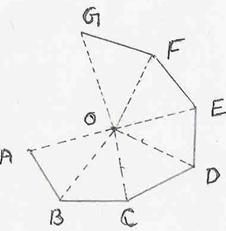1 The Polygon is made up of n triangles Polygon has n sides 2 Sum of all angles of n triangles  = n*2 right angles Sum of angles in a triangle = 2 right angles 3 Sum of angles at O = 4 right angles Angle at a point = 3600 4 Sum of all angles of n triangles = Sum of interior angles of polygon + Angle at O Construction 5 Sum of interior angles of polygon = Sum of all angles of n triangles - Angle at O Transposition of Step 4 6 = 2n right angles - 4 right angles = (2n-4)right angles Substitution (step2 and 3)

6.6.1 Corollary:

If the sides of a polygon are produced in order (Clock wise direction or anti clock wise direction), the sum of exterior angles so formed is equal

to 4 right angles. In the adjoining figure a,b,c… are the exterior angles

 Step Statement Reason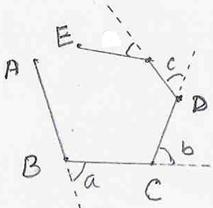1 Sum of (interior + exterior) angle at one vertex = 2 right angles angles on a straight line = 2 right angles 2 Sum of (interior + exterior) angle at n vertices  = 2n right angles Step 1 3 Sum of interior angles + sum of exterior angles  =  Sum of (interior angle + exterior angle) for n sides (vertices) Construction(figure) 4 Sum of exterior angles = Sum of (interior + exterior) angle for n sides - Sum of interior angles Transposition of Step 3 5 = 2n right angles – (2n-4) right angles (step 2 and 6.6.1 Theorem) 6 = 4 right angles

Observations:

 No Properties 1 Each interior angle of a regular polygon = (2n-4)*90/n 2 Each exterior angle of a regular polygon(x)  = 4*90/n = 360/n 3 If x is the exterior angle of a regular polygon then the number of sides(n) = 360/x

6.6.1 Problem 1:  AB, BC and CD are three consecutive sides of a regular polygon. IfBAC = 150 Find

(i) Each interior angle of the polygon

(ii) Each exterior angle of the polygon

(iii) Number of sides of the polygon

Solution:

 Since AB=BC, ABC is an isosceles triangle, henceACB =BAC = 150ABC = 1500 Thus Each interior angle = 1500 Each exterior angle = 300 Number of sides = 360/30 = 12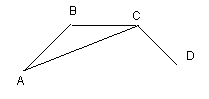6.6.1 Problem 2: Difference between the exterior angle of (n-1) sided regular polygon and the interior angle of (n+1) sided regular polygon is 90.

Find the value of n.

Solution:

1. Exterior angle of (n-1) sided regular polygon = 360/(n-1)

2. Exterior angle of (n+1) sided regular polygon = 360/(n+1)

It is given that the difference between them is 9360/(n-1) – 360/(n+1) = 9

i.e. {360(n+1) - 360(n-1)}/{(n+1)(n-1)} = 9

i.e. 720/n2-1 = 9

i.e. n2-1 = 80

i.e. n2 = 81

i.e. n = 9

The regular polygon has 9 sides.

6.6 Summary of learning

 No Points to remember 1 Polygons are figures with three or more line segments which are coplanar, non – collinear and these line segments intersect each other at their end points 2 A regular polygon is a polygon which is both equilateral and equiangular 3 An inscribed regular polygon is a regular polygon whose vertices are on the circle